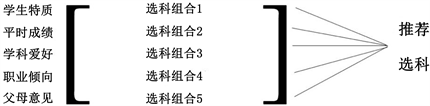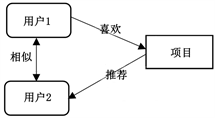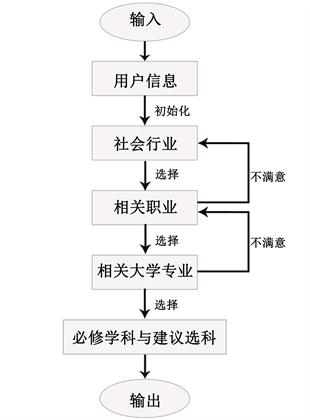# 基于模糊评价矩阵的新高考选科推荐系统New College Entrance Examination Selection Recommendation System Based on Fuzzy Evaluation Matrix

DOI: 10.12677/CSA.2020.102037, PDF, HTML, XML, 下载: 291  浏览: 627  科研立项经费支持

Abstract: With the progress of the new college entrance examination reform, it is urgent for candidates to choose the most suitable subjects for themselves. This paper focuses on the personalized selection of candidates, applies the fuzzy comprehensive evaluation method to the recommendation of the college entrance examination, uses the cooperative filtering algorithm to speed up the operation of the recommendation system of the college entrance examination, and uses the conversational interactive decision-making to recommend the subject selection according to the career vision and the university specialty. This paper designs a complete system, in which the above methods are applied in the system, so as to achieve better results than a single score selection. Finally, the fuzzy matrix is constructed through the investigation experiment in a middle school, and the first five factors affecting the subject selection are identified. Performance, interest, professional tendency, the family will, the influence weight of student’s characteristics, carry on the selection section recommendation, achieve the remarkable effect, thus better satisfy the examinee’s many aspects demand.

1. 引言

2. 理论与方法

2.1. 模糊综合评价法

2.1.1. 模糊综合评价模型的基本步骤

$B=A\cdot R=\left({a}_{1},{a}_{2},\cdots ,{a}_{n}\right)\left[\begin{array}{ccc}{r}_{11}& \cdots & {r}_{1m}\\ ⋮& \ddots & ⋮\\ {r}_{n1}& \cdots & {r}_{nm}\end{array}\right]=\left({b}_{1},{b}_{2},\cdots ,{b}_{m}\right)$ (1)

2.1.2. 确定评价指标体系Figure 1. Fuzzy selection evaluation matrix model

${a}_{j}=\frac{{c}_{j}}{\underset{i=1}{\overset{n}{\sum }}{c}_{i}}$ (2)

2.2. 基于用户的协同过滤算法Figure 2. User-based collaborative filtering algorithm

$\mathrm{cos}\theta =\frac{{\sum }_{i=1}^{n}\left({A}_{i}×{B}_{i}\right)}{\sqrt{{\sum }_{i=1}^{n}{\left({A}_{i}\right)}^{2}}×\sqrt{{\sum }_{i=1}^{n}{\left({B}_{i}\right)}^{2}}}$ (3)Figure 3. Subject selection model based on CF

2.3. 会话式根据职业倾向进行选科决策Figure 4. Conversational course selection decision flow chart

2.4. 其他部分模型

3. 实验

3.1. 数据集

3.1.1. 确定指标权重

3.1.2. 选科组合特征Table 1. Explanation and description of the questionnaireTable 2. Feature vector of each subject combination

3.2. 进行模糊综合评价选科

${r}_{ij}=\frac{{v}_{ij}}{{\sum }_{j=1}^{6}{v}_{ij}}$ (4)

bj为j学科最后的综合评分，也是隶属最后选科组合的程度，取其中综合最高的三个学科为最后的决策学科。Table 3. The average school ranking of Student Xx

$\mathrm{cos}\theta \left({X}_{x},{X}_{3}\right)=\frac{{\sum }_{i=1}^{n}\left({X}_{x}{}_{i}×{X}_{3}{}_{i}\right)}{\sqrt{{\sum }_{i=1}^{n}{\left({X}_{x}{}_{i}\right)}^{2}}×\sqrt{{\sum }_{i=1}^{n}{\left({X}_{3}{}_{i}\right)}^{2}}}$ = 0.78

$\begin{array}{c}B=\left[0.33,0.28,0.2,0.11,0.08\right]\left[\begin{array}{cccccc}1/3& 1/3& 0& 0& 0& 1/3\\ 1/3& 1/3& 1/3& 0& 0& 0\\ 1/2& 0& 1/2& 0& 0& 0\\ 1/3& 1/3& 0& 0& 0& 1/3\\ 1/3& 1/3& 0& 0& 1/3& 0\end{array}\right]\\ =\left[0.367,0.267,0.193,0,0.03,0.147\right]\end{array}$

3.3. 实验结果与分析

3.3.1 评价指标

$A=\left(\text{TP}+\text{TN}\right)/\text{All}$ (5)

3.3.2. 实验结果

3.3.3. 实验分析

4. 总结

NOTES

*通讯作者。

  逯行, 沈阳. 智能技术变革教育的基础方向与行动进路[J]. 现代教育技术, 2019, 29(11): 39-45.  曾召霞, 刘道华, 王蒙恩, 崔艺婧. 大数据时代的基础教育质量动态模糊综合评价体系建设研究[J]. 无线互联科技, 2019, 16(19): 117-118.  林丽青, 黄洁仪. 基于多层次模糊综合评价法的高职学生信息化学习满意度实证研究[J]. 吉林省教育学院学报, 2019, 35(8): 124-127.  黄斌, 杨馨宇, 吕梅. 平台类教育App评价指标体系的构建与应用研究[J]. 中国教育信息化, 2019(16): 75-81.  王佳艺. 政府信息公开绩效评估体系构建研究[D]. 黑龙江: 黑龙江大学, 2013.  孔丹. 基于平衡计分卡的商业银行绩效评价体系研究[D]: [硕士学位论文]. 武汉: 武汉理工大学, 2010.  邵淑华. 教师信息技术应用能力模糊综合评价模型[J]. 数字通信世界, 2018(11): 259-260.  李海博. 城乡结合部常规公交服务满意度评价与对策研究[D]: [硕士学位论文]. 重庆: 重庆交通大学, 2016.  林韶娟. 基于信任网络的推荐系统研究[D]: [硕士学位论文]. 上海: 复旦大学, 2012.  杜育林. 基于信息熵的高维分类型数据子空间聚类算法研究[D]: [硕士学位论文]. 汕头: 汕头大学, 2011.  李承前. 网络知识资源深层表示学习模型研究[D]: [硕士学位论文]. 上海: 东华大学, 2015.  艾静超. 基于改进协同过滤技术的个性化旅游线路推荐研究[J]. 现代电子技术, 2019, 42(23): 182-186.  陈耶拉, 耿秀丽. 基于改进协同过滤的个性化产品服务系统方案推荐[J/OL]. 计算机集成制造系统: 1-12. http://kns.cnki.net/kcms/detail/11.5946.TP.20191129.1140.002.html, 2020-01-27.  徐进波, 王寒. 生产决策管理可视化交互设计在教育信息化的应用研究[J]. 设计艺术研究, 2019, 9(2): 55-60+68.  张宇宁, 侯福均. 基于属性间交互作用指标的多属性决策方法[J]. 运筹与管理, 2018, 27(9): 1-7.  樊凌. 智慧教育背景下基于大数据的学生成绩预测模型[J]. 信息与电脑(理论版), 2019, 31(24): 223-225.  张怡彪. 新高考背景下高中生选科问题的案例分析与研究[C]//广西写作学会教学研究专业委员会. 2019年教学研究与教学写作创新论坛成果集汇编(三). 广西写作学会教学研究专业委员会: 广西写作学会教学研究专业委员会, 2019: 539-541.